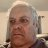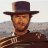# Variable starting at 1

V

#### vettett15

##### New member
Trying to setup a simple counter and ran into an issue where on the first bar of the data my "Counter10" and "Counter11" variables have an initial value of 1.

I don't understand it because since my condition10 and condition11 are 0 then i expect to get Counter10 and Counter11 which at the beginning of the day should be 0, no?

I'm looking at the SPY chart for today with the after hours turned off and at 9:30 my Counter10 and Counter11 have a value of 1 (all other values are 0)

Code:
``````input aggregationPeriod = AggregationPeriod.DAY;
input showOnlyLastPeriod = yes;

def prevPrice = open(period = aggregationPeriod)[-1];
def price = open(period = aggregationPeriod);
def PNP_HIGH = price + 0.5;
def PNP_LOW = price - 0.5;
def POMM_HIGH = price + 1.75;
def POMM_LOW = price - 1.75;

def condition1 = high >= PNP_HIGH;
def condition2 = high >= POMM_HIGH;

def condition10 = low <= PNP_LOW;
def condition11 = low <= POMM_LOW;

def Counter1 = if condition1 then Counter1 + 1 else Counter1;
def Counter2 = if condition2 then Counter2 + 1 else Counter2;

def Counter10 = if condition10 then Counter10 + 1 else Counter10;
def Counter11 = if condition11 then Counter11 + 1 else Counter11;

plot data1 = low;
plot data2 = PNP_LOW;
plot data10 = condition10;
plot data1001 = Counter10;

plot data3 = low;
plot data4 = POMM_LOW;
plot data11 = condition11;
plot data1101 = Counter11;``````

V

#### vettett15

##### New memberCreating a counter with variable input Questions 4How do you make a timeframe a variable? Questions 1